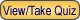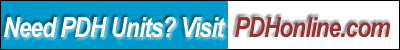Print this page

Maximum Likelihood & Gauss-Markov Parameter Estimation

Drayton D. Boozer, Ph.D, P.E.

Course Outline

The need to fit mathematical models to measured data arises often in science and engineering. Parameter estimation is a disciple that provides estimates of unknown parameters in a system or process model based on measured data. The professional analyst can use the model that results from the application of parameter estimation to explain measured data to customers in a concise, compelling way.

The course develops maximum likelihood and Gauss-Markov parameter estimators for a linear measurement model. Six basic assumptions about measurement errors that were introduced in Course G429 are used to clearly explain when to apply each estimation method. Confidence limits for the estimated parameters are developed. Finally, maximum likelihood and Gauss-Markov estimators are compared to the least squares estimator developed in Course G429.

A detailed thermal conductivity example problem is solved and discussed.

This is the second in a series of courses planned to give a working level understanding of the field of parameter estimation to practicing engineers, land surveyors, and architects.

This course includes a multiple-choice quiz at the end, which is designed to enhance the understanding of the course materials.

Learning Objective

After taking this course and successfully passing the quiz, the student will be able to:

• Confidently present and defend maximum likelihood and Gauss-Markov parameter estimation results to clients and interested parties.
• Understand when and when not to apply the maximum likelihood and Gauss-Markov estimators for a given set of measurement error assumptions.
• Understand how maximum likelihood and Gauss-Markov estimators are related to the least squares estimator presented in Course G429.
• Estimate the parameters in a linear measurement model by applying the maximum likelihood estimator to a set of measurements.
• Estimate the parameters in a linear measurement model by applying the Gauss-Markov estimator to a set of measurements.
• Estimate the unknown measurement error variance using both maximum likelihood and an alternative unbiased estimator.
• Determine confidence limits for estimated parameters for normally distributed measurement errors with known covariance matrix.
• Determine confidence limits for estimated parameters for normally distributed measurement errors with unknown variance but known correlation structure.
• Establish the basis for understanding parameter estimation techniques for nonlinear system.
• Establish the basis for extending confidence limits for individual parameter estimates to multivariate confidence regions of the estimated parameter vector for both linear and nonlinear systems.

Intended Audience

This course builds on Course G429, Linear Least Squares Parameter Estimation, to develop maximum likelihood and Gauss-Markov parameter estimators for a linear measurement model. Professional engineers, land surveyors, and architects who encounter problems where a model must be fit to measurement data will find this course useful. Both the maximum likelihood and Gauss-Markov estimators are applicable to parameter estimation problems where the measurement errors have any valid covariance structure. The maximum likelihood estimator developed in this course requires normally distributed measurement errors, whereas the Gauss-Markov estimator does not. Confidence limits are developed for the maximum likelihood parameter estimates. Professionals who anticipate the need to develop and/or communicate parameter estimation results to clients and peers should find the unified, concise presentation in these courses easy to learn and apply.

Those taking the course should have an introductory understanding of probability theory, statistics, and matrix/vector notation.

Benefit to Attendees

This course series provides professionals a sound mathematical methodology for generating, presenting and defending model-fit-to-measurement-data results to clients and other interested parties.

Course Introduction

Professional engineers, land surveyors, and architects are often asked to make customer recommendations based on a limited set of uncertain measurements of a physical system or process. Mathematical models and statistical techniques can be used to provide the theoretical foundation that enables reliable, supportable recommendations. The purpose of this course is to provide the student with the necessary understanding that enables such recommendations.

Parameters are constants found in mathematical models of systems or processes. Parameter estimation is a disciple that provides estimates of these unknown parameters in a system or process model based on measurement data. Parameter estimation is a very broad subject that cuts a broad swath through engineering and statistical inference. Because parameter estimation is used in so many different academic and application areas, the terminology can be confusing to the uninitiated.

In this course we develop maximum likelihood and Gauss-Markov estimators for a linear measurement model. The course extends the material in Course G429, Linear Least Squares Parameter Estimation.

Course Content

The course content is in a PDF file (221 KB) Maximum Likelihood & Gauss-Markov Parameter Estimation. You need to open or download above documents to study this course.

Table of Content

Introduction
Measurement Model
Statistical Assumptions for Measurement Errors
Maximum Likelihood Estimation
Estimation of Measurement Error Variance
Thermal Conductivity Example
Gauss-Markov Estimation
Comparison of Three Estimation Methods
Summary

Course Summary

This course presents maximum likelihood and Gauss-Markov parameter estimators with a focus on six basic assumptions that can be made about the measurement errors. The measurement error assumption sets for which maximum likelihood and Gauss-Markov are the appropriate estimation technique are clearly delineated. Confidence limits for the parameter estimates provide quality measures useful in communicating results to clients. A detailed thermal conductivity example problem is solved and discussed. A table is presented that concisely summarizes least squares, maximum likelihood, and Gauss-Markov estimators for a linear measurement model.

1. Web site for t distribution and associated table
http://www.itl.nist.gov/div898/handbook/eda/section3/eda3672.htm

Quiz

Once you finish studying the above course content, you need to take a quiz to obtain the PDH credits.DISCLAIMER: The materials contained in the online course are not intended as a representation or warranty on the part of PDH Center or any other person/organization named herein. The materials are for general information only. They are not a substitute for competent professional advice. Application of this information to a specific project should be reviewed by a registered architect and/or professional engineer/surveyor. Anyone making use of the information set forth herein does so at their own risk and assumes any and all resulting liability arising therefrom.